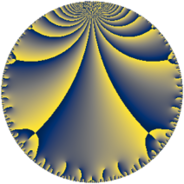# Properties

 Label 43.7.fLevel $43$ Weight $7$ Character orbit 43.f Rep. character $\chi_{43}(2,\cdot)$ Character field $\Q(\zeta_{14})$ Dimension $126$ Newform subspaces $1$ Sturm bound $25$ Trace bound $0$

# Related objects

## Defining parameters

 Level: $$N$$ $$=$$ $$43$$ Weight: $$k$$ $$=$$ $$7$$ Character orbit: $$[\chi]$$ $$=$$ 43.f (of order $$14$$ and degree $$6$$) Character conductor: $$\operatorname{cond}(\chi)$$ $$=$$ $$43$$ Character field: $$\Q(\zeta_{14})$$ Newform subspaces: $$1$$ Sturm bound: $$25$$ Trace bound: $$0$$

## Dimensions

The following table gives the dimensions of various subspaces of $$M_{7}(43, [\chi])$$.

Total New Old
Modular forms 138 138 0
Cusp forms 126 126 0
Eisenstein series 12 12 0

## Trace form

 $$126q - 7q^{2} - 7q^{3} + 725q^{4} - 7q^{5} + 244q^{6} + 2233q^{8} + 6312q^{9} + O(q^{10})$$ $$126q - 7q^{2} - 7q^{3} + 725q^{4} - 7q^{5} + 244q^{6} + 2233q^{8} + 6312q^{9} + 1955q^{10} - 985q^{11} - 455q^{12} - 4485q^{13} - 6771q^{14} + 5017q^{15} - 41935q^{16} - 10409q^{17} - 5110q^{18} - 33397q^{19} - 24507q^{20} - 5448q^{21} + 25193q^{22} - 29017q^{23} - 1885q^{24} - 2080q^{25} + 34713q^{26} - 7q^{27} + 28665q^{28} + 17353q^{29} - 290052q^{30} + 13547q^{31} + 458283q^{32} - 94801q^{33} - 191527q^{34} + 183008q^{35} + 956352q^{36} + 304607q^{38} - 381031q^{39} + 66419q^{40} + 313447q^{41} - 323228q^{43} + 281588q^{44} - 1237551q^{45} + 80948q^{46} - 180133q^{47} - 762055q^{48} - 1861754q^{49} + 999215q^{51} + 471797q^{52} + 1030011q^{53} - 92099q^{54} + 324233q^{55} - 842713q^{56} + 331037q^{57} + 849461q^{58} - 369609q^{59} + 553323q^{60} - 594727q^{61} - 886711q^{62} + 1914136q^{63} - 3250219q^{64} + 1473521q^{65} - 427559q^{66} + 122535q^{67} - 602346q^{68} + 4320057q^{69} - 1694994q^{70} - 770707q^{71} - 391202q^{72} + 476945q^{73} - 3943812q^{74} + 2061367q^{75} - 2044462q^{76} + 3016216q^{77} + 239350q^{78} + 5077268q^{79} + 2388946q^{81} + 5820437q^{82} - 1324235q^{83} + 3759618q^{84} - 4967947q^{86} - 3537894q^{87} - 7289555q^{88} - 4331495q^{89} - 12825206q^{90} + 1802976q^{91} - 12038260q^{92} + 6249341q^{94} - 5313259q^{95} + 7069598q^{96} + 788975q^{97} + 4724783q^{98} - 2216971q^{99} + O(q^{100})$$

## Decomposition of $$S_{7}^{\mathrm{new}}(43, [\chi])$$ into newform subspaces

Label Dim. $$A$$ Field CM Traces $q$-expansion
$$a_2$$ $$a_3$$ $$a_5$$ $$a_7$$
43.7.f.a $$126$$ $$9.892$$ None $$-7$$ $$-7$$ $$-7$$ $$0$$